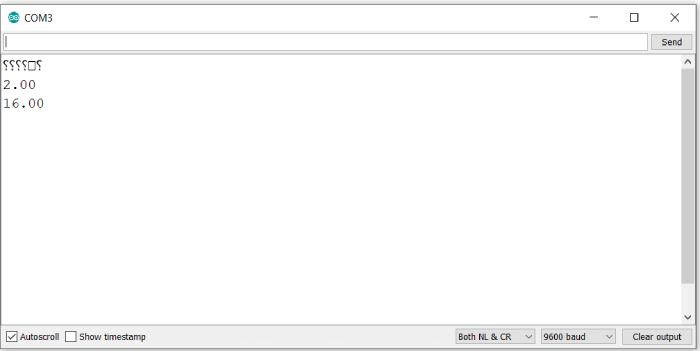# Square and Square root in Arduino

Arduino has support for several popular math functions, square and square root being among them. Let’s look at the square root first.

## Syntax

sqrt(x)

where is a number of any data type. It returns a double.

For square, you ideally shouldn’t need a separate function. You can just multiply the number by itself.

x_squared = x*x;

However, Arduino does have a separate function for calculating squares. The syntax is −

sq(x) where x is a number of any data type. This again returns a double.

## Example

The following example illustrates the use of these functions −

void setup() {
// put your setup code here, to run once:
Serial.begin(9600);
Serial.println();
float x = 4.0;
Serial.println(sqrt(x));
Serial.println(sq(x));
}

void loop() {
// put your main code here, to run repeatedly:
}

## Output

The Serial Monitor output is shown below −